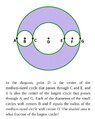# -gre.ge.3 Circles Find the shaded area as a fraction

• MHB
Gold Member
MHB
View attachment 9352

Ok this is considered a "hard" GRE geometry question... notice there are no dimensions
How would you solve this in the fewest steps?

#### Attachments

•Screenshot_20191109-122447_Docs.jpg
54 KB · Views: 41

Gold Member
MHB
Ok this is considered a "hard" GRE geometry question... notice there are no dimensions
How would you solve this in the fewest steps?
You have three circles inside. Call the radius of the largest inner circle r. Then the radius of the two smaller circles are r/2 and the radius of the big circle on the outside is 2r.

You are supposed to give the answer as a ratio so the r's eventually cancel out.

Can you finish?

-Dan

Gold Member
MHB
You have three circles inside. Call the radius of the largest inner circle r. Then the radius of the two smaller circles are r/2 and the radius of the big circle on the outside is 2r.

You are supposed to give the answer as a ratio so the r's eventually cancel out.

Can you finish?

-Dan

Kinds I know that the area of a circle is
$A=\pi r^2$

skeeter
Come on ... you can do this

Big circle - (medium circle + 2 small circles)

Gold Member
MHB
If the radius of the big circle is 2 then the total of the 3 interior radius' is 1 since they are all on the diameter of the big circle.

$\dfrac{\pi(1)^2}{\pi(2)^2}=\dfrac{1}{4}$

Kinda...like..

Gold Member
MHB
If the radius of the big circle is 2 then the total of the 3 interior radius' is 1 since they are all on the diameter of the big circle.

$\dfrac{\pi(1)^2}{\pi(2)^2}=\dfrac{1}{4}$

Kinda...like..
Check those radii again... Try calling the radius of one of the small circles to be r = 1 and give it another try. Then look at this:
Area of large circle in the center: $$\displaystyle A =\pi r^2$$
Area of the two smaller circles: $$\displaystyle 2a = 2 ( \pi (r/2)^2 ) = (1/2) \pi r^2$$
Area of the whole circle: $$\displaystyle A_{big} = \pi (2r)^2 = 4 \pi r^2$$

Sum of the area of the inner circles: $$\displaystyle \pi r^2 + (1/2) \pi r^2 = (3/2) \pi r^2$$

So what is the ratio?

-Dan

Gold Member
MHB
Check those radii again... Try calling the radius of one of the small circles to be r = 1 and give it another try. Then look at this:
Area of large circle in the center: $$\displaystyle A =\pi r^2$$
Area of the two smaller circles: $$\displaystyle 2a = 2 ( \pi (r/2)^2 ) = (1/2) \pi r^2$$
Area of the whole circle: $$\displaystyle A_{big} = \pi (2r)^2 = 4 \pi r^2$$
Sum of the area of the inner circles: $$\displaystyle \pi r^2 + (1/2) \pi r^2 = (3/2) \pi r^2$$

$\dfrac{4 \pi r^2-(3/2) \pi r^2}{4 \pi r^2}=\dfrac{8 \pi r^2-(3) \pi r^2}{8 \pi r^2}=\dfrac{5}{8}$

maybe

Last edited:
skeeter
not maybe ... 5/8 is correct

Gold Member
MHB
mahalo sorry my likes were so late

Last edited: